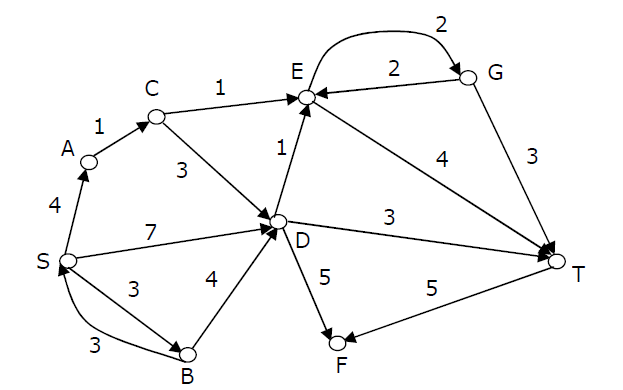Open in App
Not now

# Data Structures and Algorithms | Set 29

• Difficulty Level : Easy
• Last Updated : 13 Dec, 2022

Following questions have been asked in GATE 2012 exam.

1) The recurrence relation capturing the optimal time of the Tower of Hanoi problem with n discs is
(A) T(n) = 2T(n – 2) + 2
(B) T(n) = 2T(n – 1) + n
(C) T(n) = 2T(n/2) + 1
(D) T(n) = 2T(n – 1) + 1

Following are the steps to follow to solve Tower of Hanoi problem recursively.

```Let the three pegs be A, B and C. The goal is to move n pegs from A to C.
To move n discs from peg A to peg C:
move n-1 discs from A to B. This leaves disc n alone on peg A
move disc n from A to C
move n?1 discs from B to C so they sit on disc n```

The recurrence function T(n) for time complexity of the above recursive solution can be written as following.

T(n) = 2T(n-1) + 1

2) Consider the directed graph shown in the figure below. There are multiple shortest paths between vertices S and T. Which one will be reported by Dijkstra?s shortest path algorithm? Assume that, in any iteration, the shortest path to a vertex v is updated only when a strictly shorter path to v is discovered.(A) SDT
(B) SBDT
(C) SACDT
(D) SACET

3) Suppose a circular queue of capacity (n – 1) elements is implemented with an array of n elements. Assume that the insertion and deletion operation are carried out using REAR and FRONT as array index variables, respectively. Initially, REAR = FRONT = 0. The conditions to detect queue full and queue empty are
(A) Full: (REAR+1) mod n == FRONT, empty: REAR == FRONT
(B) Full: (REAR+1) mod n == FRONT, empty: (FRONT+1) mod n == REAR
(C) Full: REAR == FRONT, empty: (REAR+1) mod n == FRONT
(D) Full: (FRONT+1) mod n == REAR, empty: REAR == FRONT